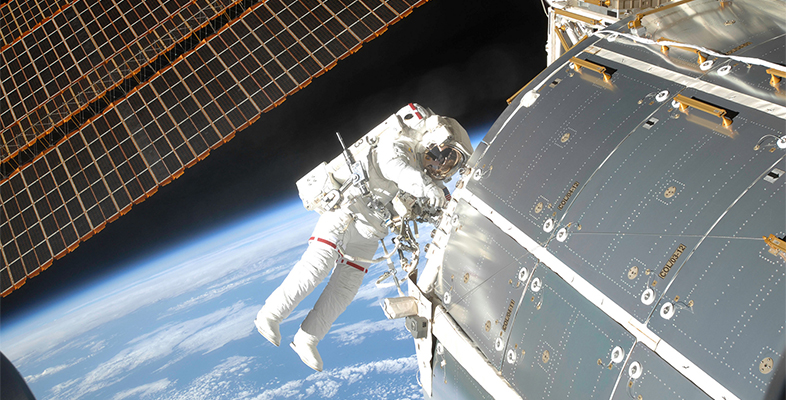Science, Maths & Technology

### Become an OU studentMicrogravity: living on the International Space Station

Start this free course now. Just create an account and sign in. Enrol and complete the course for a free statement of participation or digital badge if available.

# 3.1 How to launch a rocket

How can you launch a rocket into space? Obviously, you need something to push it upwards, so providing a force. This force is often called a ‘thrust’, which is measured in newtons (N). Burning rocket fuel to provide this force is one obvious solution. Opposing this motion, in addition to gravity, is air resistance. This is often called ‘drag’ and is also measured in N.

You then need to consider the mass of the rocket – measured in kilograms (kg) – and how long the fuel burns – measured in seconds (s). As the fuel is gradually exhausted, there is a reduction in the overall mass of the whole rocket. This is because the mass of fuel is reduced as time increases. This change of mass in time is given in kg/s.

Now complete Activity 4.

## Activity 4 Launching your own rocket

Timing: Allow approximately 25 minutes

Using the link below to simulate a rocket launch, try to overcome the gravitational ‘pull’ of the Earth – the Earth’s gravitational field. In the following simulation you can change the mass, thrust, thrust time, drag and mass change. See how far you can launch a rocket into space. [Tip: hold Ctrl and click a link to open it in a new tab. (Hide tip)]

Firstly, use the default settings where mass = 1200 kg, thrust = 25000 N, thrust time = 20 seconds, drag (off), mass change (off). Press the ‘launch’ button. What were your results?

Answer; Your rocket did not go high enough to launch the satellite. Height reached = 5 km and maximum speed = 2 m s-1

Now record what happens when you change the parameters as follows.

• Mass change = ‘off’
• Drag forces = ‘on’ (click on the left-hand button under the ‘drag forces’ option)
• Thrust time = 120 s
• Thrust = 141 200 N
• Mass = 1200 kg

This was a ‘catastrophic failure’! The unmanned rocket has exploded due to high stress. Height reached = 1 km and maximum speed = 423 m s-1

Now try Task 2 where the mass has been increased but all the other variables have been kept constant.

• Mass = 11 460 kg
• Thrust = 141 200 N
• Thrust time = 120 s
• Drag forces = ‘on’
• Mass change = ‘off’

This was a ‘partial launch’. Height reached = 21 km and maximum speed = 278 m s-1

The ‘extra challenge’ is to switch off on both drag and mass change to see how the flight of the rocket would be different. Try this now in Task 3. Again, we have only changed one variable, keeping the others constant.

• Mass = 11 460 kg
• Thrust = 141 200 N
• Thrust time = 120 s
• Drag forces = ‘on’
• Mass change = ‘on’ (click on the left-hand button under this option)

Again, this is a partial launch. This time the rocket reached a maximum height of 67 km, with a maximum speed of 793 m -1.

Now see if you can achieve ‘successful deployment’ of the satellite.

#### Discussion

To do this, you need to use the following parameters.

• Mass = 7511 kg
• Thrust = 122 400 N
• Thrust time = 140 s
• Drag forces = ‘off’
• Mass change = ‘on’

There are many other online rocket simulations. Here are a few to explore if you have time.

So now, when people say ‘It’s not rocket science’, you can reply by saying that it is!